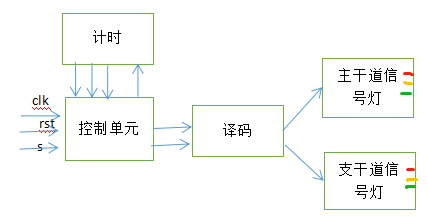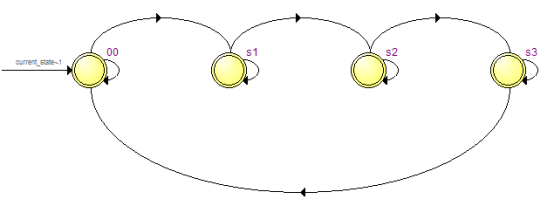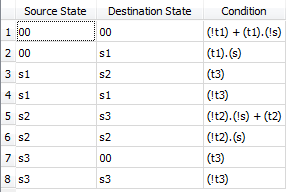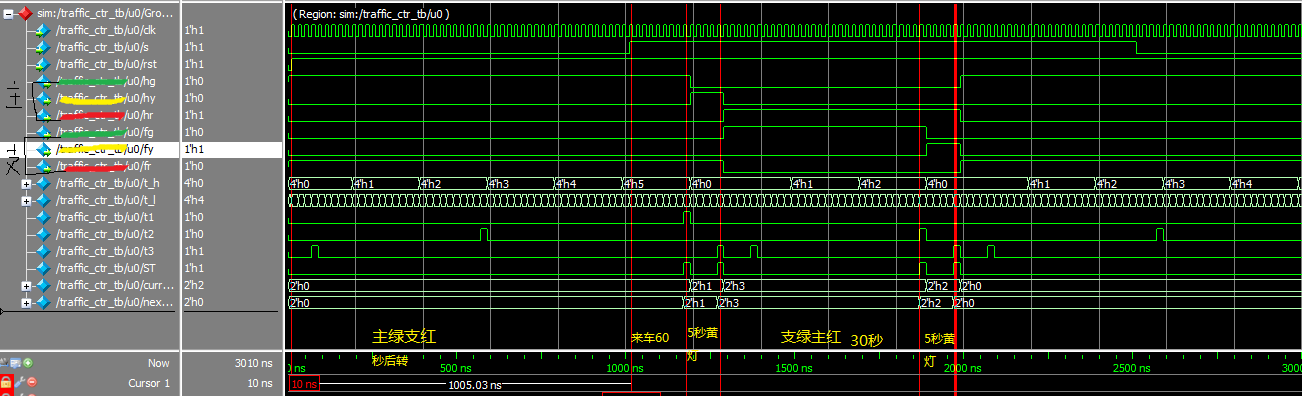# 交通信号灯控制系统的Verilog实现

（1）一般情况下保持主干道通畅，主干道绿灯亮、支干道红灯亮，并且主干道绿灯亮时间不少于60秒。
（2）主干道绿灯亮超过60秒，且支干道有车时，主干道红灯亮，支干道绿灯亮，但支干道亮灯时间不超过30秒。
（3）每次主干道或支干道绿灯变红灯时，黄灯先亮5秒。
1.逻辑抽象，明确输入输出。

2.确定系统框图S0状态 主干道绿灯亮支干道红灯亮，此时若支干道有车等待通过，而且主干道绿灯已亮足规定的时间t1，控制器发出状态转换信号St，输出从状态S0转换到S1 （转换条件：绿灯亮够60s且检测到支干道有车即 t1&&s=1

S1状态 主干道黄灯亮，支干道红灯亮，进人此状态，黄灯亮足规定的时间t3时，控制器发出状态转换信号St，输出从状态S1转换到S2。 （转换条件：黄灯亮够5s就转换状态即 t3=1

S2状态 支干道绿灯亮，主干道红灯亮，若此时支干道继续有车，则继续保持此状态，但支干道绿灯亮的时间不得超过t2，否则控制单元发出状态转换信号St，使输出转换到S3状态。若此时支干道没有车，则控制单元立即发出状态转换信号St，使输出转换到S3状态。
（转换条件：支干道绿灯亮够30s或者检测到支干道没车即 t2+（~s）=1

S3状态 支干道黄灯亮，主干道红灯亮，此时状态与S1状态持续的时间相同均为t3，时间到时，控制器发出St信号，输出从状态S3回到S0状态。对上述S0、S1、S2和S3四种状态按照格雷码进行编码分别为00，01，11和10。
/控制状态机/
always@(posedge clk or negedge rst)
begin
if(!rst)
current_state<=2’d0;
else
current_state<=next_state;
end

always@(s,current_state,t1,t2,t3)
begin
case(current_state)
s0:	begin
next_state=(t1&&s)?s1:s0;
ST=(t1&&s)?1'b1:1'b0;
end
s1:	begin
next_state=(t3)?s2:s1;
ST=(t3)?1'b1:1'b0;
end
s2:	begin
next_state=(t2||~s)?s3:s2;
ST=(t2||~s)?1'b1:1'b0;
end
s3:	begin
next_state=(t3)?s0:s3;
ST=(t3)?1'b1:1'b0;
end
endcase
endalways@(posedge clk or negedge rst)   //计时模块
begin
if(!rst)
{t_h,t_l}<=8'd0;
else if(ST)             //控制信号有效说明灯的颜色改变重新计数
{t_h,t_l}<=8'd0;
else if((t_h==4'd5)&(t_l==4'd9))   //判断是否计数到60
begin
{t_h,t_l}<={t_h,t_l};
end
else if(t_l==4'd9)   //判断个位是否计数满，是则十位加一
begin
t_h<=t_h+1'b1;
t_l<=4'd0;
end
else begin
t_h<=t_h;
t_l<=t_l+1'b1;
end
end
assign t3=(t_h==4'd0)&(t_l==4'd4);   //主干道绿灯计数时间
assign t2=(t_h==4'd2)&(t_l==4'd9);	 //	支干道绿灯计数时间
assign t1=(t_h==4'd5)&(t_l==4'd9);   //黄灯计数时间


always@(current_state)
begin
case(current_state)
s0:	begin
{hg,hy,hr}=3'b100;  //主干道绿灯
{fg,fy,fr}=3'b001;  //支干道红灯
end
s1:	begin
{hg,hy,hr}=3'b010;  //主干道黄灯
{fg,fy,fr}=3'b001;  //支干道红灯
end
s2:	begin
{hg,hy,hr}=3'b001;  //主干道红灯
{fg,fy,fr}=3'b100;  //支干道绿灯
end
s3:	begin
{hg,hy,hr}=3'b001;  //主干道红灯
{fg,fy,fr}=3'b010;  //支干道黄灯
end
endcase
end05-06
04-1111-207522
10-191993
05-17
10-171万+
04-232万+
10-251万+
09-031760
08-061875
01-259871
06-054166
03-27
06-181576点击重新获取扫码支付余额充值